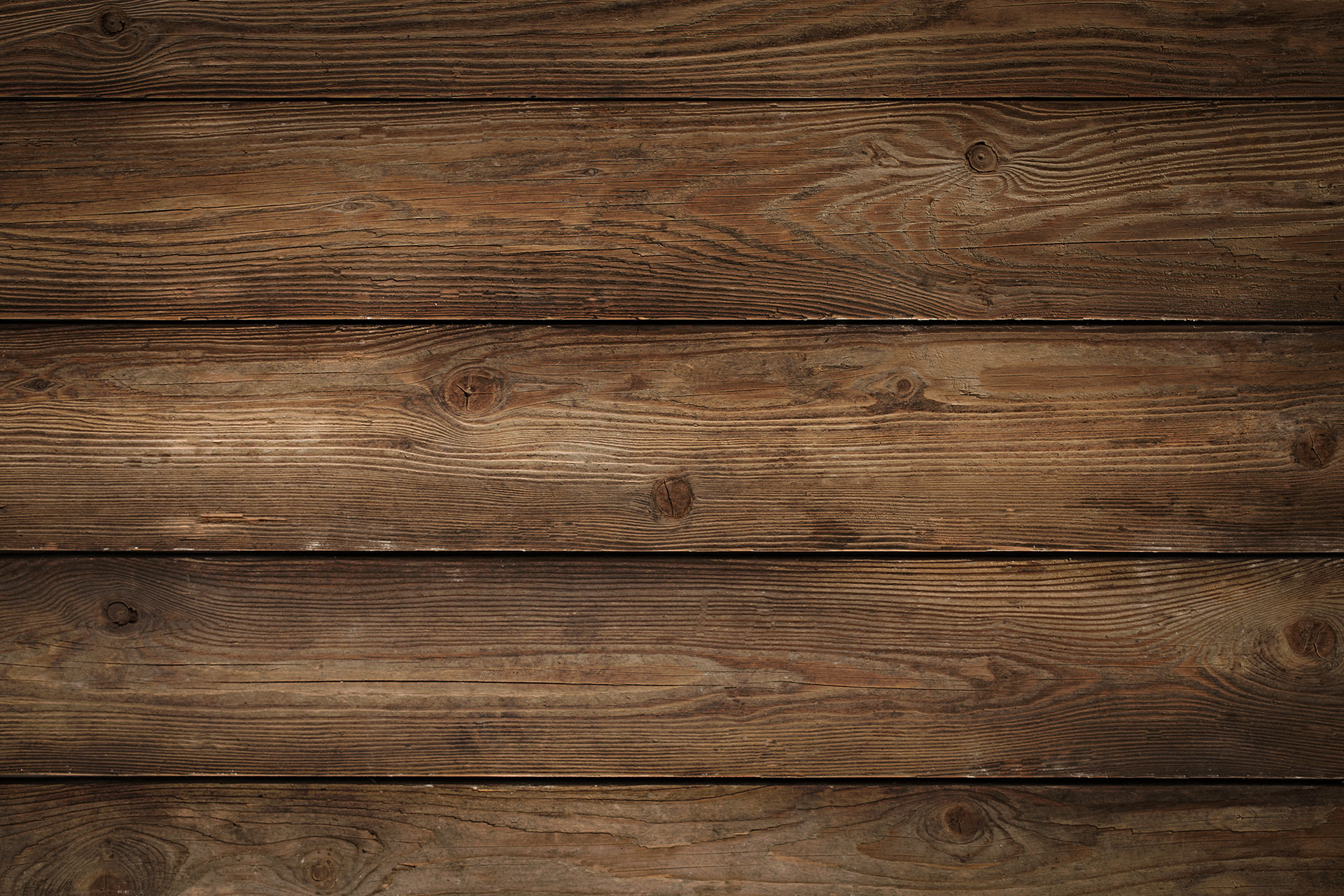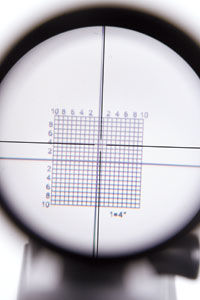top of pageSearch
• Ken Kraushaar

# How to use a CollimatorIf you're like me, you probably have heard of, or at least used a collimator to adjust the scopes on your rifle. it's a tool that uses a spud to hold a piece of glass that has a grid or it's own reticle (crosshair) and projects that reticle or a grid on the scope's field of view, allowing you to adjust the scope so it's "close" and will get you on paper.

However, the drawback is that, even at 100 yards, you will most likely be low, due to the amount of drop a bullet has, even at a relatively short distance of 100 yards. Ultimately what the Collimator does, is align the field of view of your scope, to the axis of the bore of your barrel, meaning the image you see through your scope would be the same as if you were looking through the bore of your rifle.

To save some ammunition and time when zeroing your rifle, you'll need to know one of two things: either the mid-range trajectory of the bullet you're shooting at the range you want to zero your rifle in at, or the drop of the bullet at the range you want to sight the rifle in at, both of which would be in inches. Then, you need to do some math.

Here is the mathematical equation for finding out the amount of adjustment in Minute of Angle (inches) you will need to make in order to get your bullet within 1 or 2 inches of the intended zero, before you ever shoot your rifle at the range:

A= 4m+h/r/100

h= the distance in inches above the bore diameter

m= midrange trajectory in inches at "R"

R= Range in yards

A= Angle in minutes between the collimator and the line of sight.

for this equation we will assume we have a "known" midrange trajectory. in days past this was included in reloading guides or it was data provided by ammunition companies on the box. we'll assume a range of 300 yards, with a scope height of 1.5" above the center bore with 1/4 MOA adjustments, and a starting velocity of 3000fps.

ex: 30 caliber 150 grain spire point bullet

Range @muzzle @100yards @200yards @ 300 yards

Velocity (fps) 3000fps 2730 2470 2230

Energy (ftlbs) 3000ftlbs 2483 2033 1656

Drop (inches) 0 2.09" 9.48" 23.62"

Midrange Traj 0 .52" 2.37" 5.90"

so we can see that the midrange trajectory is 5.90". as a side note, you can figure out the midrange trajectory by dividing the drop by 4. for example 23.60 / 4 = 5.9 which is the listed midrange trajectory for this bullet.

so we now know that:

h=1.5"

m=5.90"

R=300

so we can plug it into the equation:

4(5.90")+(1.5") / 300/100 which would then be 23.6"+1.5" / 3 which then becomes 25.1 / 3= 8.366666667 or rounded to 8. now multiply 8 by the number of clicks (4) or 8*4=32 clicks "up" to bring the adjustment close to a zero at 300 yards.

even at 100 yards, if we plug in the numbers, 4(.52)+(1.5) = (2.08")+(1.5") or 3.58" / 1 which then equals 3.58, or roughly 4 inches low, so we multiply by our clicks 4*4=16 clicks "up."

There is a separate equation for figuring out the angle without the midrange trajectory, which simply uses the drop, which most ballistics programs can now provide.

that equation is A=100(D+h) / R

so again: D=23.62" h=1.5" and R=300 yards.

A= 100(23.62+1.5) / 300 -> A= 100(25.12") / 300 -> A=2512 /300 -> A=8.37 or A=8 (rounded.)

now multiply the adjustment by 4 clicks and you get 32clicks "up" for the adjustment, which is the same as our last equation.

By doing some simple math, we can then figure out our adjustment before we ever go to the range, which saves us time and ammunition which could be used to dial in the rifle.

please note: when using handloads, you also will need to use a chronograph to find your true velocity, and the use of a ballistics calculator to figure out the drop for the bullet at the range in order for this math to work correctly.

hopefully this is useful, and happy shooting!

#Technicalinfo

262 views

See All
bottom of page